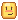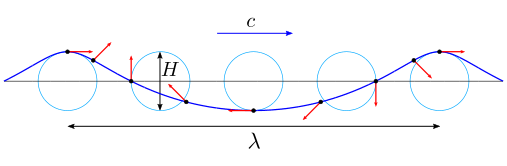# Trochoidal Wave (Gerstner Wave) Formulae?

## Wikipedia formulae;

https://wikimedia.org/api/rest_v1/media/math/render/svg/c11e5bcd5db126d232b7503bde64065aa3b89cf5

## My Formulae

``````local X = function(a, b, t)
return a + e ^ k * b / k * math.sin(k * (a + c * t))
end

local Y = function(a, b, t)
return b - e ^ k * b / k * math.cos(k * (a + c * t))
end
``````

# Thank You! I will appreciate the help.

3 Likes

Hello again!The formula seems correct. As far as variables go, the Wikipedia page you linked explains all of them pretty well. All the formal names and needed sub-calculation formulas are included as well. Take a look at Description of classical trochoidal wave category.

You can already see that c represents speed, more accurately phase speed. Phase often, and in this case also, means horizontal shift. This value determines how fast your wave will move by x-axis. Speed is a scalar quantity that describes magnitude on position change (between the position part was previously in to the current position in coordinate system). Formula is written in the article too, which is

``````                                   c^2 = g/k
``````

What does g mean? Gravitational acceleration, which is approximately 9.8 m/s2 on Earth (varies slightly), and 196.2 stud/s2 in Roblox world by default.

k is wave number, about which you can read here, and λ (Greek letter lambda) is a sign for wave length in physics. You will meet it a lot when you start going in depth about waves.

``````                                   k = 2π / λ
``````(image by Kraaiennest, Trochoidal wave - Wikipedia)

What about e? e is usually Euler constant. Also known as Euler number, it is, similar to pi (π), an infinite number, and one of the most important numbers in mathematics. Mr. Leonard Euler, Swiss mathematician, calculated it using a particular developed algorithm. It is equal to around 2.71828 and more imformation about it is available here..

I really suggest you explore those Wikipedia links further, because they hide the answers to most of your questions.

Good luck!

EDIT @xDeltaXen example? By function being used by all constants, do you mean a function that uses all involved variables, including those variables that are used to calculate the necessary coefficients? Since those variables are assembled (hopefully this is the right choice of words), they can be calculated using other equations. Finally, it all comes down to configurations (what is desired wave length, speed are we looking for etc.).

You can simply (not always simply of course) calculate k, for example, from desired wave length. Wave length can be either custom number, or calculated using

``````                                  λ = v / f
``````

with f being frequency. You can continuously calculate values this way.

A good example? Trochoidal waves (Gerstner waves) are used to calculate all the necessary components of artificial progressive waves. Probably the best examples are Roblox simulated waves, but unfortunately, I don’t currently have time to try to create them. What I can do is show you this code with made up values. You can get the idea about how waves are changing:

`````` local c = 3
local k = 2
local e = 2.71828

local function getX(a, b, t)
return a + e ^ k * b / k * math.sin(k * (a + c * t))
end

local function getY(a, b, t)
return b - e ^ k * b / k * math.cos(k * (a + c * t))
end

local part = Instance.new("Part")
part.Name = "sample"
part.Anchored = true

local current = Vector3.new()

while (true) do
local _part = part:Clone()
_part.CFrame = CFrame.new(getX(3, 8, os.clock()), 10, getY(7, 15, os.clock())) + current
_part.Parent = workspace
current += Vector3.new(c,0,0)
game:GetService("RunService").Heartbeat:Wait()
end
``````
6 Likes

Would you be able to give an example of the function being used with all constants?

1 Like

Thank you so much! Really appreciated!

2 Likes

Hi, I want to make a wave system for my game, and I’m having some trouble figuring out the math.
So the example you have provided works fine, but it is only 2-dimensional. What would need to change for a 3-dimensional system?

To allow floating things on the waves, I need a function that takes in an X and Z coordinate and returns a Y- coordinate (height at this point). I can’t figure out how to do this.
I looked at the Wikipedia page but I don’t understand what the parameters “a” and “b” mean. They seem to shift the wave and allow for sharper peaks?

Previously I was using this function, from this thread:

``````function GerstnerWave(SamplePosition ,Wavelength, Direction, Steepness, Gravity, SampleTick)
local k = (2 * math.pi) / Wavelength
local a = Steepness/k
local d = Direction.Unit
local w = math.sqrt(Gravity / k) -- Speed at which waves move
local f = k * d:Dot(Vector2.new(SamplePosition.X,SamplePosition.Z)) - w * SampleTick
local cosF = math.cos(f)

--Displacement Vectors
local dX = (d.X * (a * cosF))
local dY = a * math.sin(f)
local dZ = ( d.Y * (a * cosF))
return Vector3.new(dX, dY, dZ)
end
``````

But I found it to be very slow and I didn’t understand the math behind it. So I decided to try searching for better alternatives. Is this function really bad or is this as optimized as it can be for 3-dimensional waves?

2 Likes